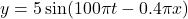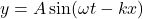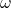Question

A plane progressive
the expression
in time, ys
where you
progressivo ware is no presented by
(At + A
y- 5 sin
in metre, t es in time the doplicensel
Calculate
the amplitude of the wave.​

1.minhkhoi

Amplitude, A = 5 m

Explanation:

Let a progressive wave is given by equation :…..(1)

The general equation of a progressive wave is given by :….(2)

Here,

A is the amplitude of the waveis the angular frequency

k is propagation constant

We need to find the amplitude of the wave.

If we compare equations (1) and (2), we find that the amplitude of the given plane progressive wave is 5 m.